# Schur's theorem

This article describes a result related to the sectional curvature of a Riemannian manifold

This result is valid in all dimensions

## Statement

Let$(M,g)$ be a Riemannian manifold of dimension at least 3. Then the following are equivalent:

• For every point$p \in M$, the sectional curvature is constant across all tangent planes at$p$.
• The sectional curvature is constant (that is, the metric is a constant-curvature metric)

## Proof

The idea behind the proof of this result is as follows. The second condition clearly implies the first, so what we essentially need to show is that the first implies the second.

For this, we convert the given condition on sectional curvature to a condition purely in terms of the Riemann curvature tensor.

### Obtaining a scalar multiple relationship

We start with the assumption that the sectional curvature is a scalar function, or in other words, that at each point, the sectional curvature is constant for all tangent planes.

Recall that the sectional curvature of the vector space with basis$X$ and$Y$ is given by: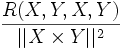$\frac{R(X,Y,X,Y)}{||X \times Y||^2}$

where the denominator is the cross product. Rewriting this in terms of the metric tensor, we get: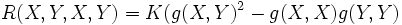$R(X,Y,X,Y) = K (g(X,Y)^2 - g(X,X)g(Y,Y)$

By polarization, we can now show that: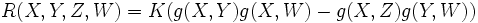$R(X,Y,Z,W) = K(g(X,Y)g(X,W) - g(X,Z)g(Y,W))$

Let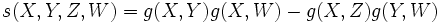$s(X,Y,Z,W) = g(X,Y)g(X,W) - g(X,Z)g(Y,W)$. Then we have:$R = Ks$

where, according to our assumptions,$K$ is a function of the point alone, viz$K$ is a scalar function.

### Applying the second Bianchi identity

The second Bianchi identity states that the cyclic summation:$\nabla_XR (Y,Z) + \nabla_YR(Z,X) + \nabla_ZR(X,Y) = 0$

Applying it to this situation, we get:$\sum \nabla_X s(Y,Z) + \sum (XK) s(Y,Z) = 0$

Since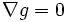$\nabla g = 0$, the product rule tells us that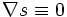$\nabla s \equiv 0$. Hence we get: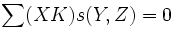$\sum (XK) s(Y,Z) = 0$

### Using the high dimension

If the tangent space at$p \in M$ has dimension at least 3, then given any vector$X$, do the following:

• Pick vectors$Y$ and$Z$ such that$X,Y,Z$ are linearly independent at$p$
• Using the definition of$s$, observe that the linear independence of$X,Y,Z$ at$p$ implies the linear independence of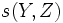$s(Y,Z)$,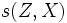$s(Z,X)$ and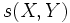$s(X,Y)$ at$p$.
• Thus, conclude that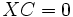$XC = 0$ at$p$.